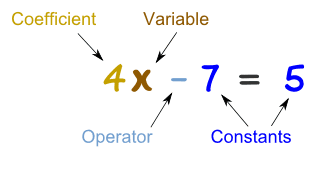Parts of an Expression
3 years ago
schaefh
Save
Edit
Host a game
Live GameLive
Homework
Solo Practice
Practice10 QuestionsShow answers
• Question 1
30 seconds
Q. The answer when you add 2 or more numbers is called the
sum
difference
product
quotient
• Question 2
30 seconds
Q. The _______ of 6 and 8 is 48.
sum
difference
product
quotient
• Question 3
30 seconds
Q. 5 is the _________ of 20 and 4.
sum
difference
product
quotient
• Question 4
30 seconds
Q. The answer when you subtract 2 or more numbers is called the ______.
sum
product
difference
quotient
• Question 5
30 seconds
Q. The number you see before a variable (Example: 6z, the 6 is the ________)
coefficient
variable
term
product
• Question 6
30 seconds
Q. A symbol for a number we don't know yet. This is usually a letter.
coefficient
sum
variable
term
• Question 7
30 seconds
Q. A number, variable or product is called a ________.
term
coefficient
variable
product
• Question 8
30 seconds
Q. A number on its own that has a fixed value.
constant
coefficient
term
variable
• Question 9
30 seconds
Q. How many coefficients are in 12x + 7y - 2
1
2
3
4
• Question 10
30 seconds
Q. What are the variables in the following expression: 3z + 4x - 1 + 7y